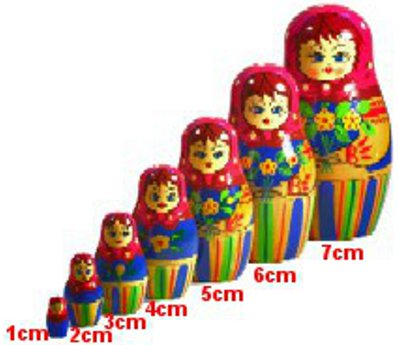# Range And Quartiles

Have you ever heard someone say that they have trouble shopping for clothes to fit them because they are too tall or too short or too fat or too slim? Why don't stores don't stock the whole range of clothes sizes to suit everyone? Obviously, stores will stock the sizes which match their customers' sizes so they can make a profit and not carry too much stock. How do store managers decide what sizes to stock? The answer is they use statistics. For example, the Inter-quartile Range is a statistical calculation used by marketing people to instruct clothing and shoe manufacturers as to the range of clothing and shoe sizes which are most likely to sell because they cater for the "middle half" of the population.

## Did You Know That...?

According to the Guinness World Records, the world's tallest man alive (Sultan Kosen from Turkey) is 251 centimetres tall, and the world's shortest man (Chandra Bahadur Dangi from Nepal) is 53 centimetres tall.

## Range

The Range is the difference between the lowest score and the highest score.

## Example One - Range

What is the height range between the world's tallest living man and the world's shortest man?

 Answer: Range = highest score – lowest score = 251 – 53 = 198 cm

## Example Two - Quartiles and Inter-Quartile RangeFor the set of Russian dolls above, calculate the 1st Quartile, the Median, the 3rd Quartile and the Inter-quartile Range.

To calculate quartiles, the data must be ordered from smallest to largest first.

1st Quartile - This is the score below which one quarter of the data lies. It is also called the Lower Quartile or Q1. In the image of the 7 Russian Maryoshka Dolls above, the 1st Quartile refers to the height of 2nd doll or 2cm.

Median - This is the score below which one half of the data lies. It is also called Q2. In the image, the 1st Quartile refers to the height of the 4th doll or 4cm.

3rd Quartile - This is the score below which three quarters of the data lies. It is also called the Upper Quartile or Q3. In the image, the 3rd Quartile refers to the height of the 6th doll or 6cm.

Interquartile Range - This is the difference between the 1st Quartile and the 3rd Quartile, that is, the "middle half" of the population. It is also called IQR.

IQR = 6cm – 2cm = 4cm.

## Example Three - Quartiles and IQR

We cannot always use a small set of easy numbers such as the doll heights in example two. Here, we will calculate the same Q1, Median and Q3, but using rules that are better suited to the large quantity of data that is more common in statistics.

The number of scores is called "n". In the dolls' example, n = 7 dolls' heights.

 1st Quartile = (n + 1) / 4 = (7 + 1) / 4 = 2nd doll = 2cm Median = (n + 1) / 2 = (7 + 1) / 2 = 4th doll = 4cm 3rd Quartile = (n + 1) / 4 × 3 = (7 + 1) / 4 × 3 = 6th doll = 6cm IQR = Q3 – Q1 = 6 – 2 = 4cm

## Example Four - Quartiles and IQR (Odd Sample Size)Find the Q1, Median, Q3 and IQR of these 11 athletes whose American shoe sizes are shown.

Ordered Data (smallest feet to largest feet) - Sizes 7, 7, 8, 9, 9, 10, 11, 11, 11, 12, 13

n = 11

 1st Quartile = (n + 1) / 4 = (11 + 1) / 4 = 3rd athlete = Size 8 Median = (n + 1) / 2 = (11 + 1) / 2 = 6th athlete = Size 10 3rd Quartile = (n + 1) / 4 × 3 = (11 + 1) / 4 × 3 = 9th athlete = Size 11 IQR = Q3 – Q1 = Size 11 – Size 8 = A difference of 3 shoe sizes

If these athletes are representative of all their customers, the shoe manufacturers will make the majority of their shoes to suit people between Size 8 and Size 11.

## Example Five - Quartiles and IQR (Even Sample Size)Find the Q1, Median, Q3 and IQR of these 12 women whose European clothing sizes are shown.

Ordered Data (slimmest to fattest) - Sizes 32, 32, 33, 33, 34, 35, 36, 37, 38, 39, 39, 40